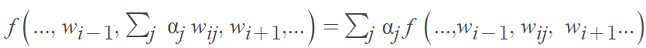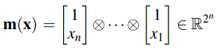# Multilinear Function: Definition & Examples

A multilinear function (also called a multilinear form) is linear in each variable separately (i.e. linear in all its arguments). In other words, it is a linear function of each variable k when all the other variables are assigned fixed values. They are polynomials of order n but with a maximum order of one for each variable .

The end result is that a quantity varies proportionally with several variables, k. Bilinear functions (k = 2) and trilinear functions (k = 3) are special cases.

## Formal Definition of a Multilinear Function

Let’s say we had the following function:
f: W1 * … * Wk → V
Where W1, …, Wk V are vector spaces over a field 𝔽.
It is a multilinear function if:For any w1W1, w2W2wnWN .
In other words, it is multilinear if it is homogenously linear in each of its arguments, k, separately.

We can also define a multilinear function f with matrices and the Kronecker product ⊗ :

f(x) = αT m(x),
where α = [α1 … α2n]T ∈ ℝ2n is a coefficient row vector andis a column vector of monomial.

## Multilinear Functions of Vectors

A function of one variable is linear if both of the following statements are true:

• f (x + y) = f(x) + f(y)
• f (cx) = cf (c).

We can build on this statement to define multilinear functions of several vectors. A function of several vectors u, v, … is multilinear if :

• f (u1 + u2, v,…) = f (u1, v,…) + (u2, v,…),
• f (cu, v, …) = cf(u, v,…)
• f (u, v1 + v2,…) = f (u1, v1,…) + (u, v2, …),
• f (u, cv, …) = cf(u, v,…)

Multilinear functions of several vectors can easily be built using cross products and dot products. For example: u, v, wu × (v × w).

## References

 Pangalos, G., Eichler, A., & Lichtenberg, G. (2015). Hybrid
multilinear modeling and applications. In Simulation
and Modeling Methodologies, Technologies and Applications (pp. 71-85). Springer, Cham.
 1 Multilinear and alternating functions. 21-801: Algebraic Methods in Combinatorics Multilinear notes. Retrieved May 3, 2021 from: http://www.borisbukh.org/AlgMethods14/multilinear_notes.pdf